batgrbproduct analysis for trigger=907950

## batgrbproduct Analysis for Trigger=907950

### Notes:

• 1) No attempt has been made to eliminate the non-significant decimal places.
• 2) This batgrbproduct analysis is done of the amount of data for this trigger downloaded to date.
• 3) This batgrbproduct analysis is using the flight position for the mask-weighting calculation.
```======================================================================
BAT GRB EVENT DATA PROCESSING REPORT
Process Script Ver: batgrbproduct v2.46
Process Start Time (local time): Wed Jun 12 20:34:33 EDT 2019
Host Name: bat1
======================================================================

Trigger: 00907950      Segment: 000

BAT Trigger Time: 581968165.056   [s; MET]
Trigger Stop: 581968168.128   [s; MET]
UTC: 2019-06-11T17:49:01.998020    [includes UTCF correction]

BAT
RA: 324.769471588208   Dec: -56.0820139162483  [deg; J2000]

Catalogged Source?: NO
Point Source?:      YES
GRB Indicated?:     YES    [ by BAT flight software ]
Image S/N Ratio:    8.07
Image Trigger?:     NO
Rate S/N Ratio:     9.8488578017961  [ if not an image trigger ]
Image S/N Ratio:    8.07

Flight Position:   [ source = BAT ]
RA: 324.769471588208   Dec: -56.0820139162483  [deg; J2000]

Ground Position:   [ source = BAT pre-slew burst ]
RA: 324.696060030405   Dec: -56.0706137552963  [deg; J2000]
{ 21h 38m 47.1s , -56d 04' 14.2" }
+/- 2.97664290058799 [arcmin] (estimated 90% radius based on SNR)
+/- 2.37649465272453 [arcmin] (formal 1-sigma fit error)
SNR: 6.40274047879776

Angular difference between Flight and Ground position is 2.551598 arcmin

Partial Coding Fraction: 0.65625    [ including projection effects ]

Duration
T90: 40.3639999628067 +/- 30.5642460239305
Measured from: -0.0279998779296875
to: 40.336000084877     [s; relative to TRIGTIME]
T50: 8.19599997997284 +/- 53.8370901378916
Measured from: 1.62800014019012
to: 9.82400012016296     [s; relative to TRIGTIME]

Fluence
Peak Flux  (peak 1 second)
Measured from: 0.544000029563904
to: 1.5440000295639     [s; relative to TRIGTIME]
Total Fluence
Measured from: -0.939999938011169
to: 63.8240000009537     [s; relative to TRIGTIME]

Band 1     Band 2     Band 3     Band 4
15-25      25-50     50-100    100-350     keV
Total     0.122745   0.236078   0.124688   0.027448
0.073995   0.076206   0.067610   0.060689 [error]
Peak      0.005686   0.045861   0.038199   0.001728
0.009643   0.011089   0.009410   0.008185 [error]

[ fluence units of on-axis counts / fully illuminated detector ]

======================================================================

=== Fluence/Peak Flux Summary ===

Model    Band1    Band2    Band3    Band4    Band5    Total
15-25    25-50   50-100  100-350   15-150   15-350   keV
Fluence   Power-law 6.48e-08 9.09e-08 9.45e-08 1.80e-07 3.07e-07 4.30e-07   erg/cm2
Error 3.38e-08 3.55e-08 6.09e-08 2.58e-07 1.44e-07 3.11e-07   erg/cm2
Peak flux Power-law 2.21e-01 2.62e-01 2.24e-01 3.27e-01 8.23e-01 1.03e+00   ph/cm2/s
Error 1.16e-01 8.93e-02 7.08e-02 2.06e-01 2.49e-01 3.05e-01   ph/cm2/s

Fluence   Cutoff-PL 4.57e-08 1.17e-07 9.75e-08 1.65e-08 2.75e-07 2.79e-07   erg/cm2
Error 4.59e-08 5.39e-08 7.26e-08 1.39e-07 1.33e-07 2.66e-07   erg/cm2
Peak flux Cutoff-PL 1.07e-01 3.00e-01 2.84e-01 5.67e-02 7.41e-01 7.48e-01   ph/cm2/s
Error 1.32e-01 1.23e-01 9.75e-02 1.48e-01 2.61e-01 2.88e-01   ph/cm2/s

Fluence        Band 1.76e-07 6.87e-07 7.11e-07 1.11e-06 1.97e-06 2.70e-06   erg/cm2
Error 7.56e-08 1.39e-07 1.87e-07 -------- 4.13e-07 --------   erg/cm2
Peak flux      Band -------- -------- -------- -------- -------- --------   ph/cm2/s
Error -------- -------- -------- -------- -------- --------   ph/cm2/s
```
```
=== Time-averaged spectral analysis ===

Model Ph_index Ph_90err Epeak  Epeak_90err Norm     Nomr_90err chi2   dof
PL    1.944    0.763    -      -           5.15e-04 2.53e-04   55.250 57
CPL  -0.364    1.079      46.7    0.0      8.96e-03 0.00e+00   54.090 56

=== 1-s peak spectral analysis ===

Model Ph_index Ph_90err Epeak  Epeak_90err Norm     Nomr_90err chi2   dof
PL    1.223    0.460    -      -           6.98e-03 2.03e-03   44.280 57
CPL  -1.685    4.521      68.9  114.9      1.49e-01 3.90e+01   41.020 56

Model      : spectral model: simple power-law (PL) and cutoff power-law (CPL)
Ph_index   : photon index: E^-{Ph_index} (minus sign is not included in the index)
Ph_90err   : 90% error of the photon index
Epeak      : Epeak in keV
Epeak_90err: 90% error of Epeak
Norm       : normalization at 50 keV in PL model and at 1 keV in CPL model
Nomr_90err : 90% error of the normalization
chi2       : Chi-Squared
dof        : degree of freedom
```
```
=== T90/T50 calculation in the 50-300 keV band (BATSE band) using 64 ms lc ===

T90 in the 50-300 keV band: 5.632000 sec.
T50 in the 50-300 keV band: 2.880000 sec.
```
```
=== Table of the duration information ==

Value   tstart    tstop
T100   64.764   -0.940   63.824
T90    40.364   -0.028   40.336
T50     8.196    1.628    9.824
Peak    1.000    0.544    1.544
```

### Image

#### Pre-slew 15-350 keV image (Event data; bkg subtracted)```#   RAcent  DECcent POSerr  Theta    Phi     Peak Cts     SNR  Name
324.6961 -56.0706 0.0300   35.4   -7.1       0.7336     6.4  TRIG_00907950
------------------------------------------

Foreground time interval of the image:
-0.940  38.645 (delta_t =  39.585 [sec])
Background time interval of the image:
-239.056  -0.940 (delta_t = 238.116 [sec])
```

### Notes:

• 1) All plots contain as much data as has been downloaded to date.
• 2) The mask-weighted light curves are using the flight position.
• 3) Multiple plots of different time binning/intervals are shown to cover all scenarios of short/long GRBs, rate/image triggers, and real/false positives.
• 4) For all the mask-weighted lightcurves, the y-axis units are counts/sec/det where a det is 0.4 x 0.4 = 0.16 sq cm.
• 5) The verticle lines correspond to following: green dotted lines are T50 interval, black dotted lines are T90 interval, blue solid line(s) is a spacecraft slew start time, and orange solid line(s) is a spacecraft slew end time.
• 6) Time of each bin is in the middle of the bin.

#### 1 s binning

##### From T0-310 s to T0+310 s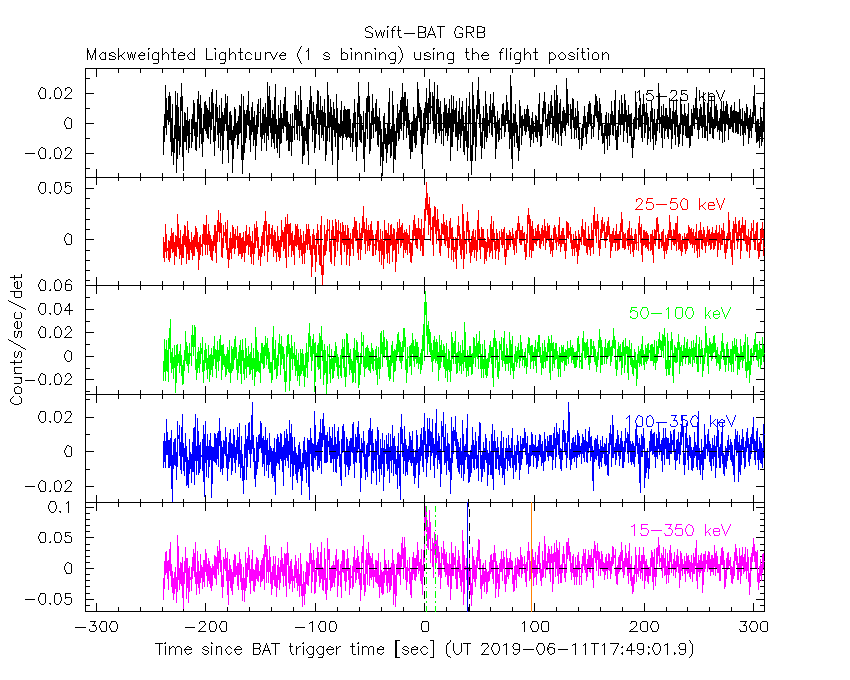##### From T100_start - 20 s to T100_end + 30 s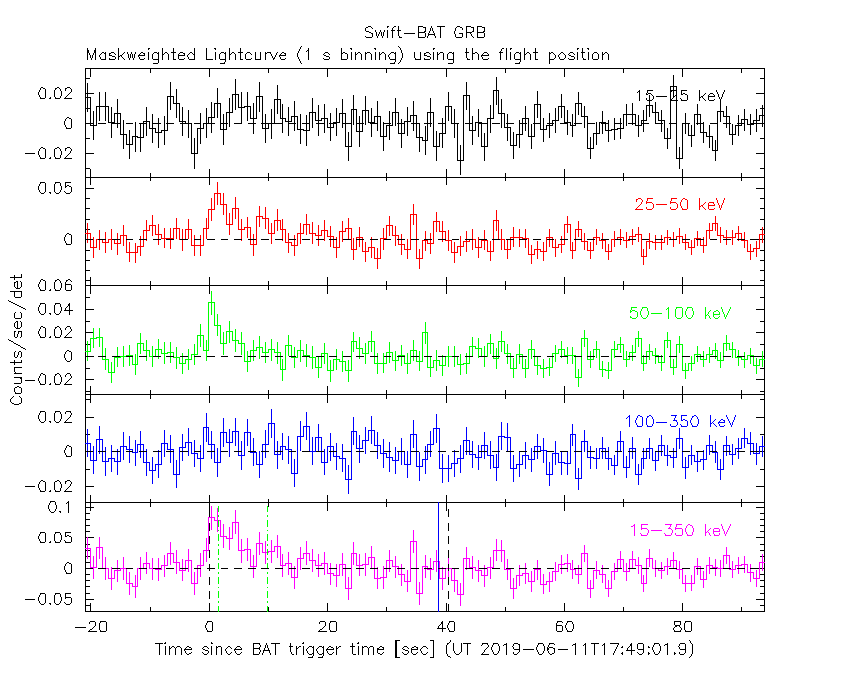##### Full time interval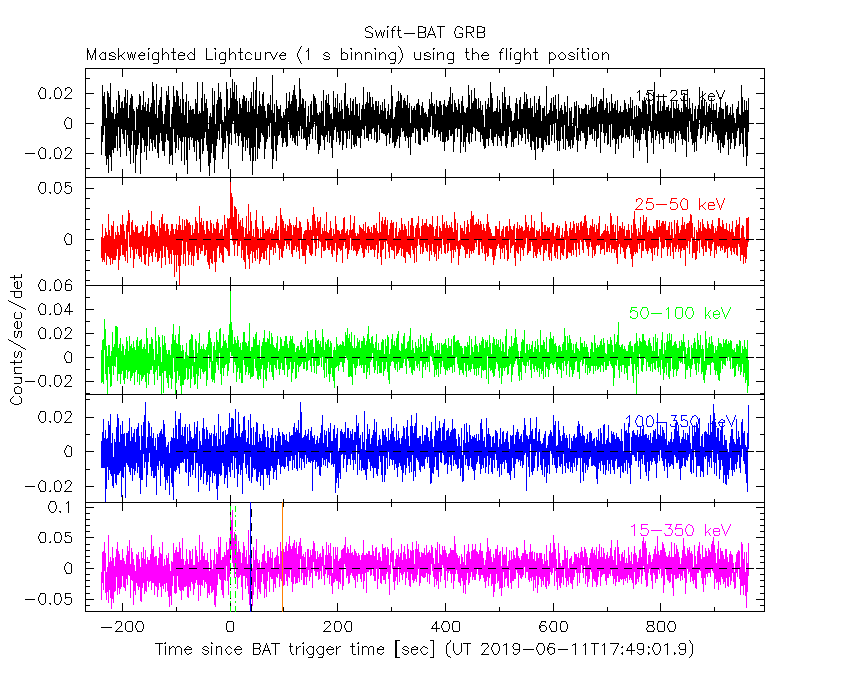#### 64 ms binning

##### Full time interval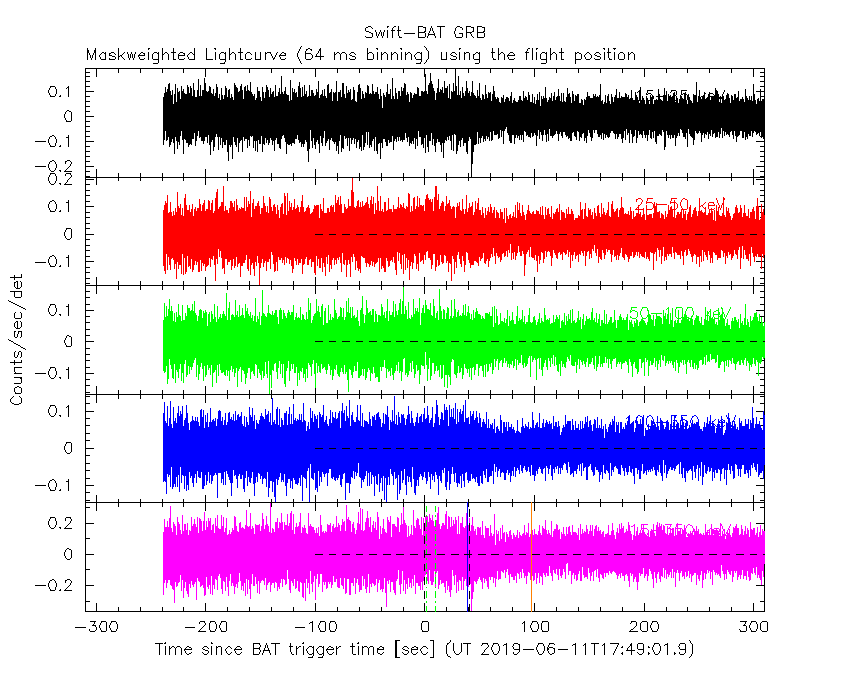##### From T100_start to T100_end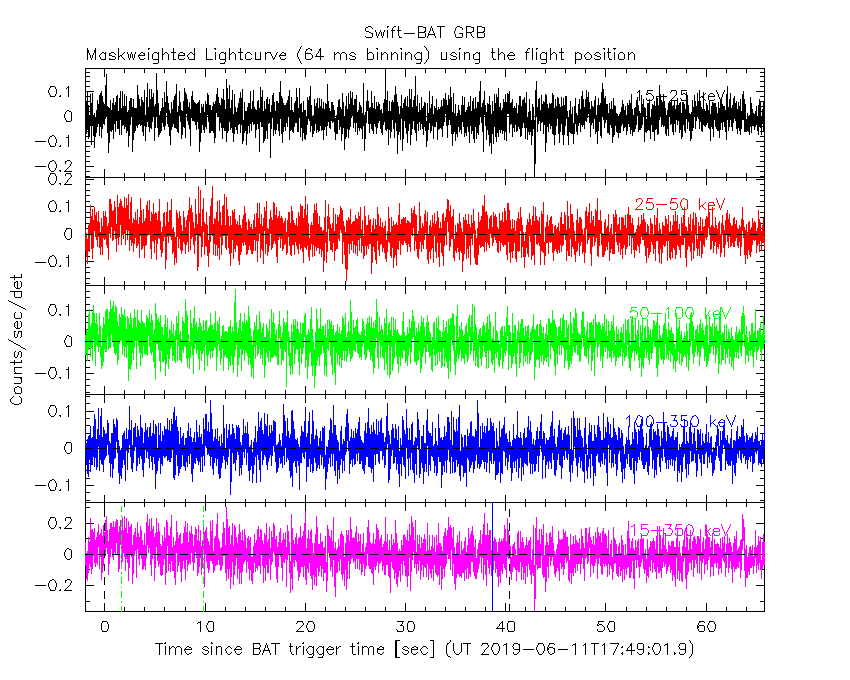##### From T0-5 sec to T0+5 sec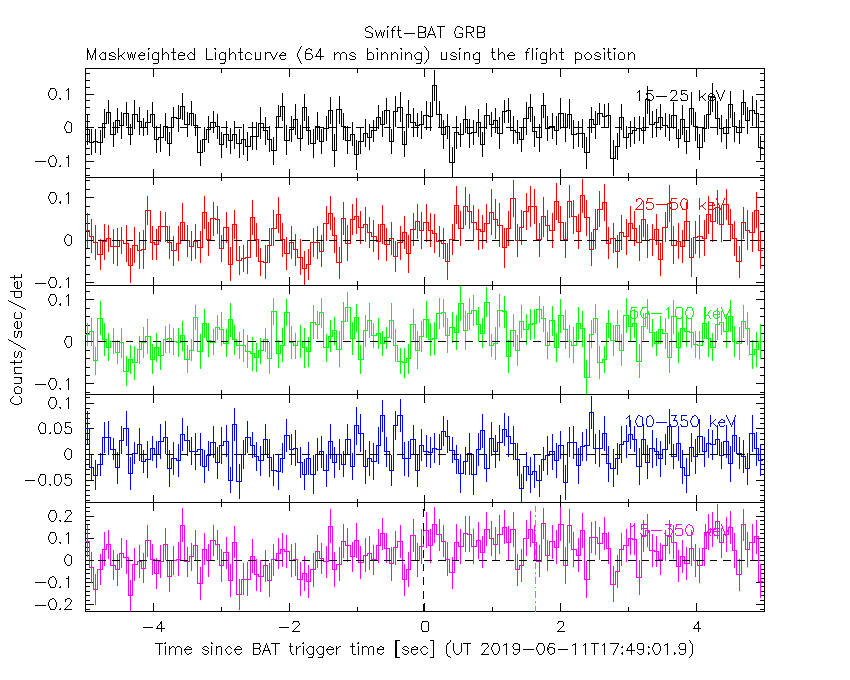#### 16 ms binning

##### From T100_start-5 s to T100_start+5 s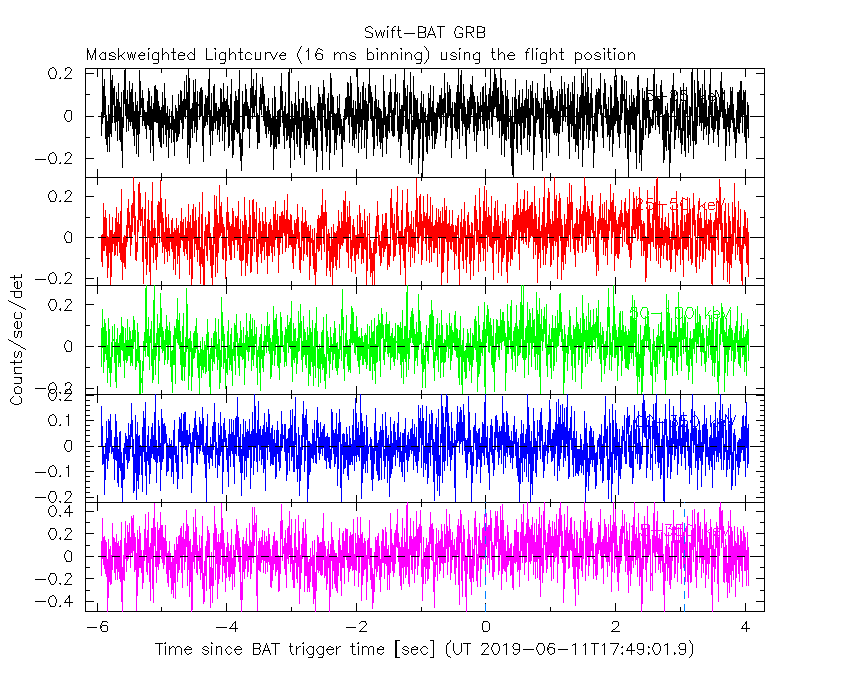#### 8 ms binning

##### From T100_start-3 s to T100_start+3 s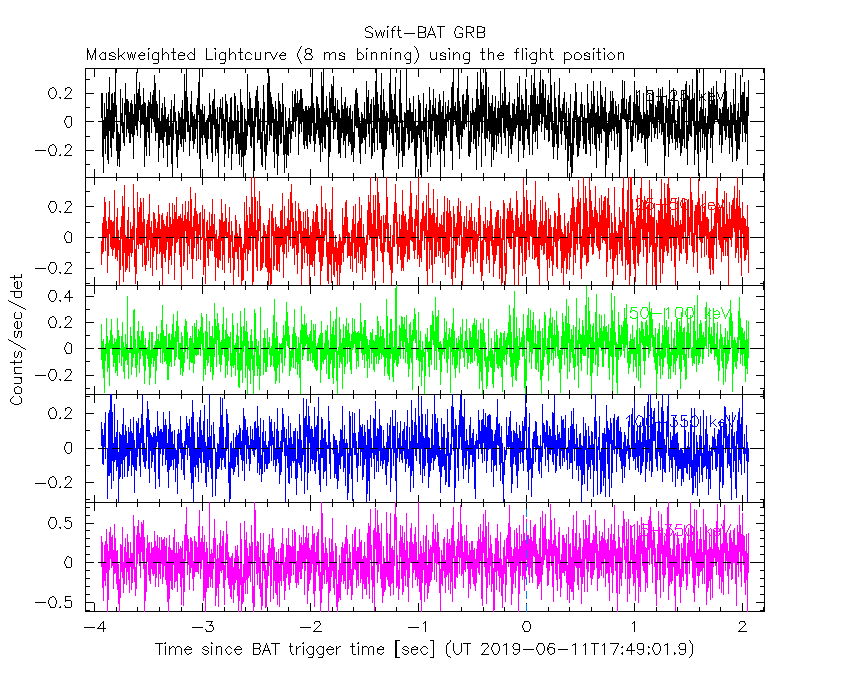#### 2 ms binning

##### From T100_start-1 s to T100_start+1 s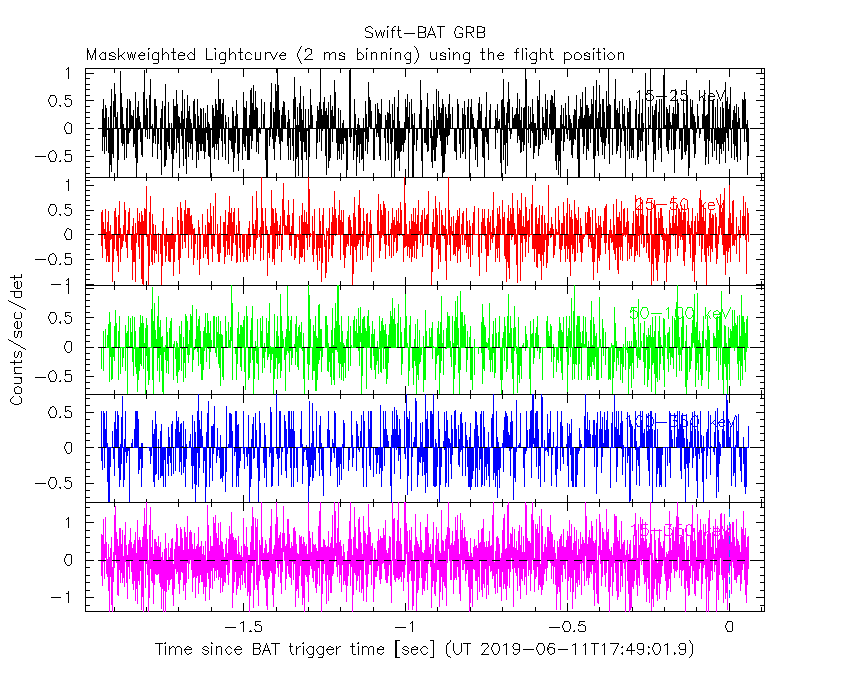#### SN=5 or 10 sec. binning (whichever binning is satisfied first)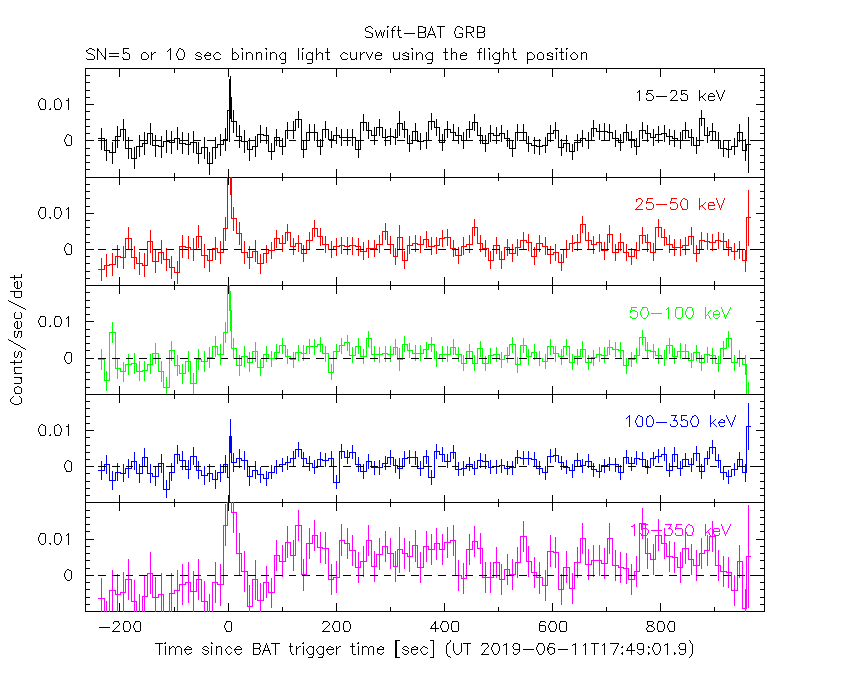#### SN=5 or 10 sec. binning (T < 200 sec)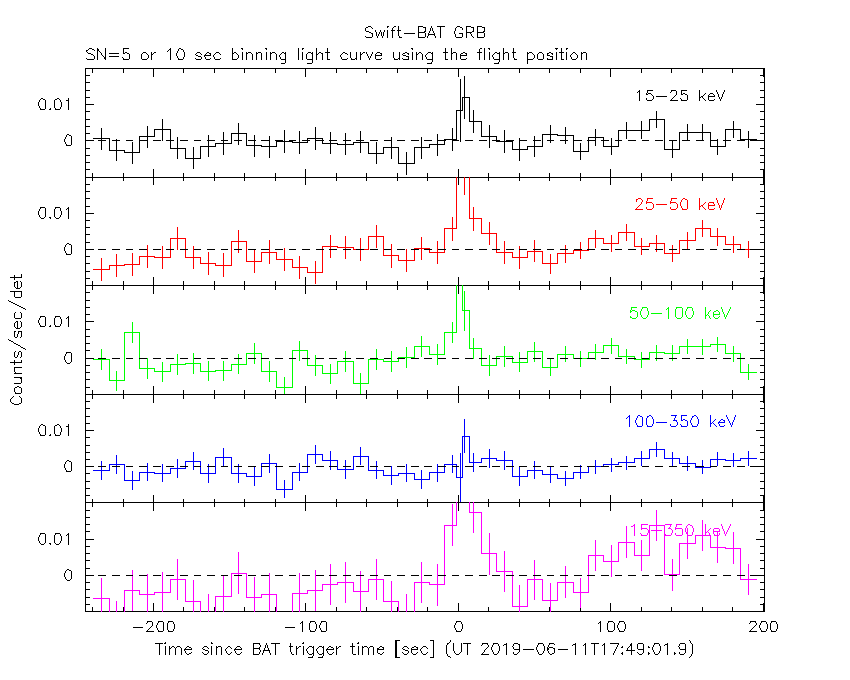### Notes:

• 1) The fitting includes the systematic errors.
• 2) For long bursts, a spectral fit of the pre-slew DRM will introduce some errors in both a spectral shape and a normalization if the PHA file contains a lot of the slew/post-slew time interval.
• 3) For fits to more complicated models (e.g. a power-law over a cutoff power-law), the BAT team has decided to require a chi-square improvement of more than 6 for each extra dof.
• 4) For short bursts (T90<1sec), the specrtum is also fit with Blackbody, OTTB, and Double Blackbody.

#### Time averaged spectrum fit using the pre-slew DRM

##### Power-law model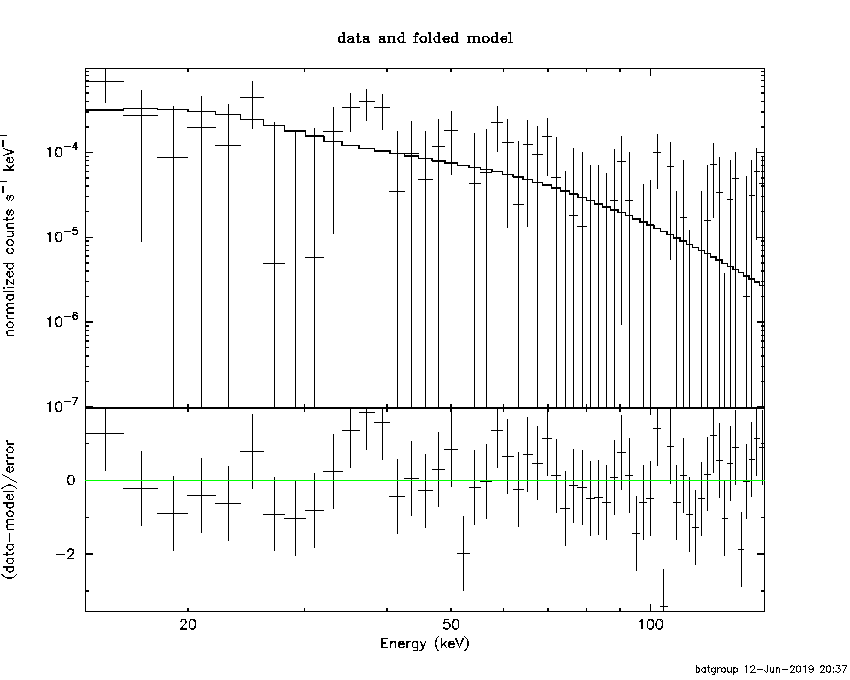```Time interval is from   -0.940 sec. to   63.824 sec.

Spectral model in power-law:
------------------------------------------------------------
Parameters  : value       lower 90%   higher 90%
Photon index: 1.94429     ( -0.658168   0.866937 )
Norm@50keV  : 5.15346E-04    ( -0.000263653   0.00024247 )
------------------------------------------------------------
#Fit statistic : Chi-Squared =          55.25 using 59 PHA bins.
# Reduced chi-squared =         0.9693 for     57 degrees of freedom
# Null hypothesis probability =   5.409997e-01

Photon flux (15-150 keV)    in 64.76 sec: 7.53873E-02 ( -0.0290652  0.0290693 ) ph/cm2/s
Energy fluence (15-150 keV)             : 3.07087e-07 ( -1.39155e-07  1.49096e-07 ) ergs/cm2

```
##### Cutoff power-law model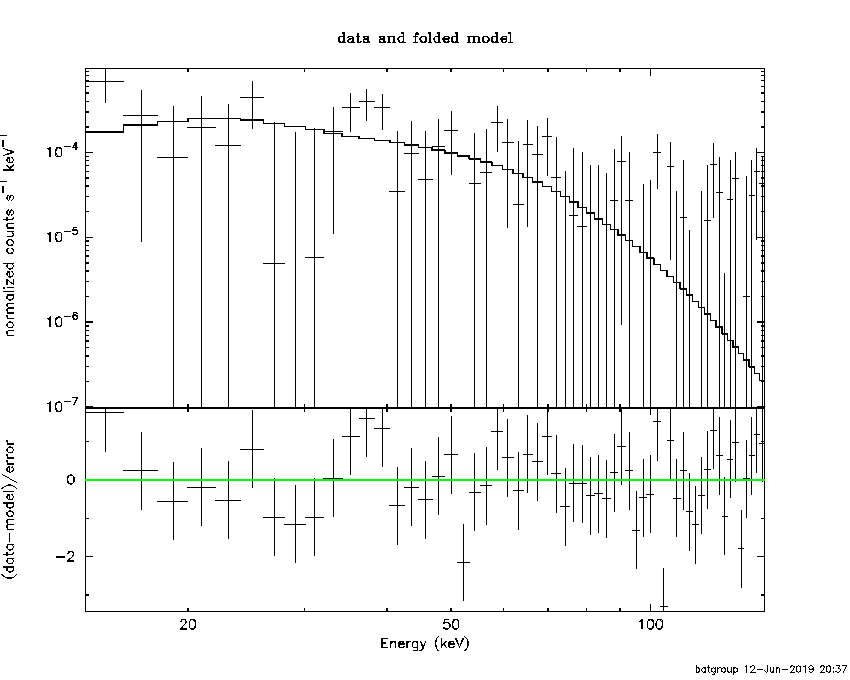```Time interval is from   -0.940 sec. to   63.824 sec.
Spectral model in the cutoff power-law:
------------------------------------------------------------
Parameters  : value       lower 90%   higher 90%
Photon index: -0.363962     ( 0.514045   2.6723 )
Epeak [keV] : 46.6596      (   )
Norm@50keV  : 8.96017E-03    (     )
------------------------------------------------------------
#Fit statistic : Chi-Squared =          54.09 using 59 PHA bins.
# Reduced chi-squared =         0.9659 for     56 degrees of freedom
# Null hypothesis probability =   5.475640e-01

Photon flux (15-150 keV)    in 64.76 sec: 6.94353E-02 ( -0.0309555  0.0306967 ) ph/cm2/s
Energy fluence (15-150 keV)             : 2.747e-07 ( -1.16225e-07  1.50323e-07 ) ergs/cm2

```
##### Band function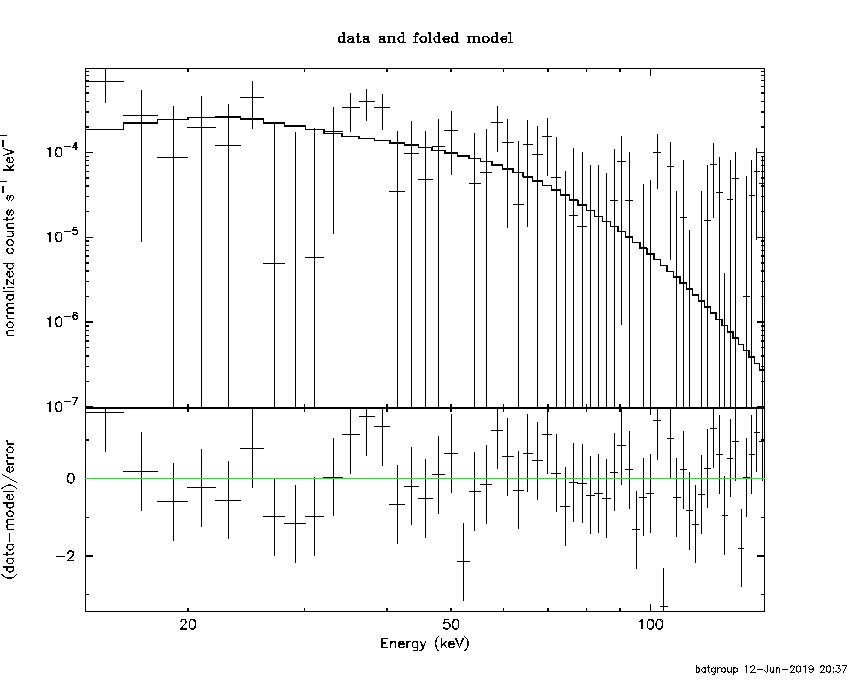```Time interval is from   -0.940 sec. to   63.824 sec.

Spectral model in the Band function:
------------------------------------------------------------
Parameters  : value       lower 90%   higher 90%
alpha       : 0.279305   ( -2.99606   2.78702 )
beta        : -10.00000    ( 10   1.87494e-11 )
Epeak [keV] : 46.6672      ( -17.5584 479.431 )
Norm@50keV   : 8.13095E-03    ( -0.00303928   4.18166 )
------------------------------------------------------------
#Fit statistic : Chi-Squared =          54.10 using 59 PHA bins.
# Reduced chi-squared =         0.9836 for     55 degrees of freedom
# Null hypothesis probability =   5.091598e-01

Photon flux (15-150 keV)    in 64.76 sec:  (    ) ph/cm2/s
Energy fluence (15-150 keV)             : 1.96837e-06 ( -1.96792e-06  -4.10021e-07 ) ergs/cm2

```
##### Single BB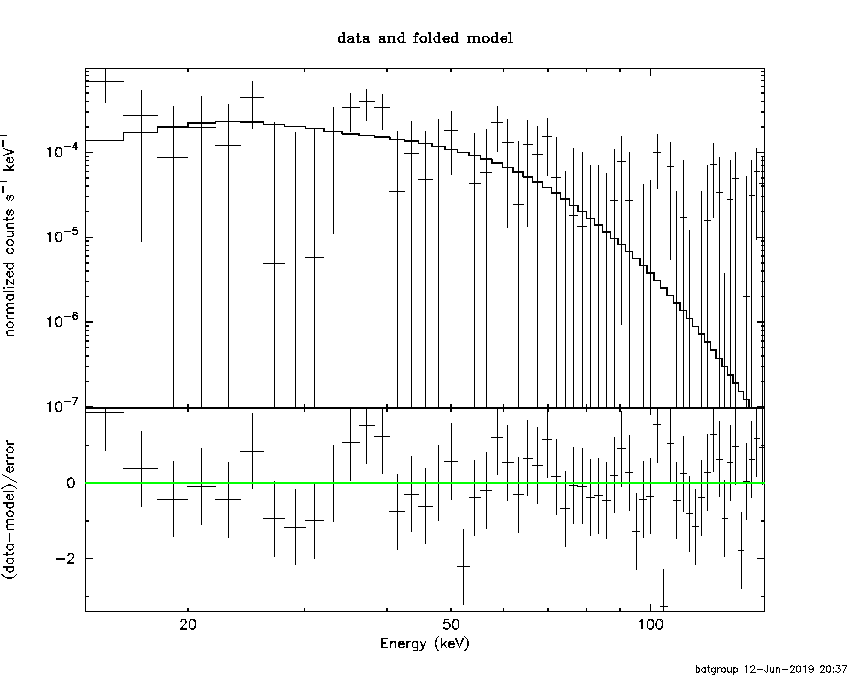```
Spectral model blackbody:
------------------------------------------------------------
Parameters  : value    Lower 90%  Upper 90%
kT [keV]    : 11.9475  (-3.70316  5.13647)
R^2/D10^2   : 1.98536E-02  (-0.0198536   0.0565501)
(R is the radius in km and D10 is the distance to the source in units of 10 kpc)
------------------------------------------------------------
#Fit statistic : Chi-Squared =          54.08 using 59 PHA bins.
# Reduced chi-squared =         0.9488 for     57 degrees of freedom
# Null hypothesis probability =   5.851843e-01

Energy  Fluence     90% Error
[keV]   [erg/cm2]   [erg/cm2]
15- 25  3.880e-08   2.561e-08
25- 50  1.215e-07   7.716e-08
50-150  1.040e-07   7.953e-08
15-150  2.643e-07   1.677e-07
```
##### Thermal bremsstrahlung (OTTB)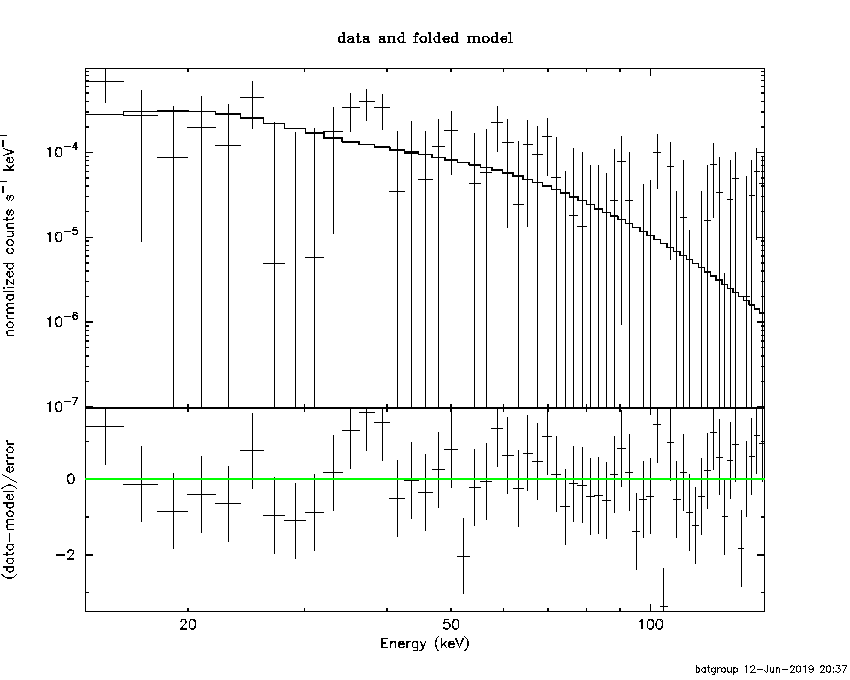```

Spectral model: thermal bremsstrahlung
------------------------------------------------------------
Parameters  : value         Lower 90%    Upper 90%
kT [keV]    : 73.2259       (-45.9736      -73.1007)
Norm        : 0.371118       (-0.163704     0.300433)
------------------------------------------------------------
#Fit statistic : Chi-Squared =          54.68 using 59 PHA bins.
# Reduced chi-squared =         0.9593 for     57 degrees of freedom
# Null hypothesis probability =   5.626976e-01

Energy  Fluence     90% Error
[keV]   [erg/cm2]   [erg/cm2]
15- 25  6.194e-08   3.957e-08
25- 50  9.972e-08   6.369e-08
50-150  1.324e-07   1.171e-07
15-150  2.940e-07   2.025e-07
```
##### Double BB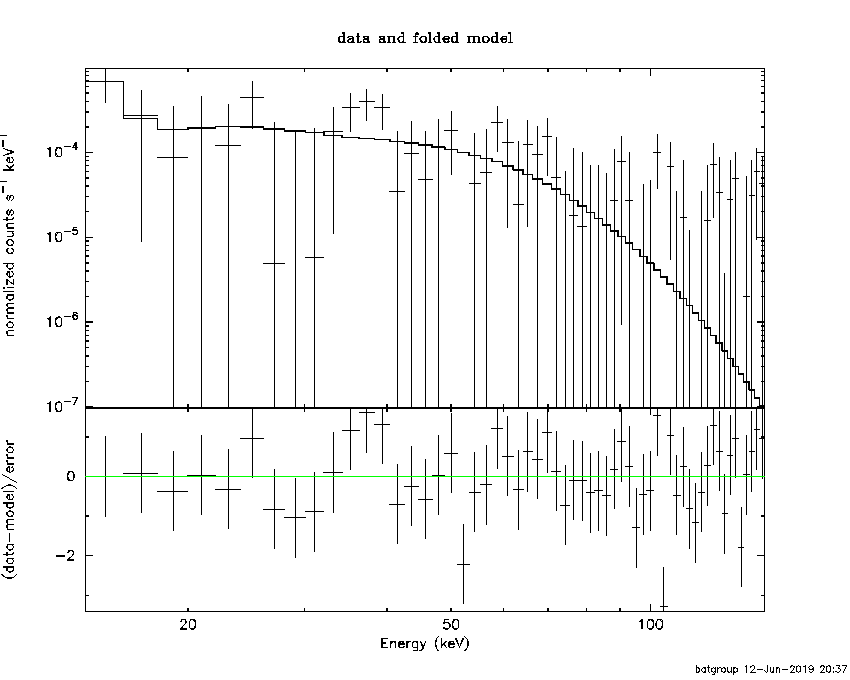```R1^2/D10^2  : 2.15946E+06        (-2.2865e+06      -2.28655e+06)
kT2 [keV]   : 12.7998        (-3.89923      5.81192)
R2^2/D10^2  : 1.45865E-02    (-0.0111256   0.0175659)
------------------------------------------------------------
#Fit statistic : Chi-Squared =          54.08 using 59 PHA bins.
#Fit statistic : Chi-Squared =          50.61 using 59 PHA bins.
#Fit statistic : Chi-Squared =          50.33 using 59 PHA bins.
# Reduced chi-squared =         0.9833 for     55 degrees of freedom
# Reduced chi-squared =         0.9202 for     55 degrees of freedom
# Reduced chi-squared =         0.9152 for     55 degrees of freedom
# Null hypothesis probability =   5.096314e-01
# Null hypothesis probability =   6.428446e-01
# Null hypothesis probability =   6.532263e-01

Energy  Fluence     90% Error
[keV]   [erg/cm2]   [erg/cm2]
15- 25  0.000e+00   0.000e+00
25- 50  0.000e+00   0.000e+00
50-150  0.000e+00   0.000e+00
15-150  0.000e+00   0.000e+00
```

#### Peak spectrum fit

##### Power-law model```Time interval is from    0.544 sec. to    1.544 sec.

Spectral model in power-law:
------------------------------------------------------------
Parameters  : value       lower 90%   higher 90%
Photon index: 1.22313     ( -0.469736   0.450292 )
Norm@50keV  : 6.98445E-03    ( -0.0020295   0.00202625 )
------------------------------------------------------------
#Fit statistic : Chi-Squared =          44.28 using 59 PHA bins.
# Reduced chi-squared =         0.7768 for     57 degrees of freedom
# Null hypothesis probability =   8.906520e-01

Photon flux (15-150 keV)    in 1 sec: 0.822639 ( -0.247769  0.250295 ) ph/cm2/s
Energy fluence (15-150 keV)             : 7.0401e-08 ( -2.13432e-08  2.15747e-08 ) ergs/cm2

```
##### Cutoff power-law model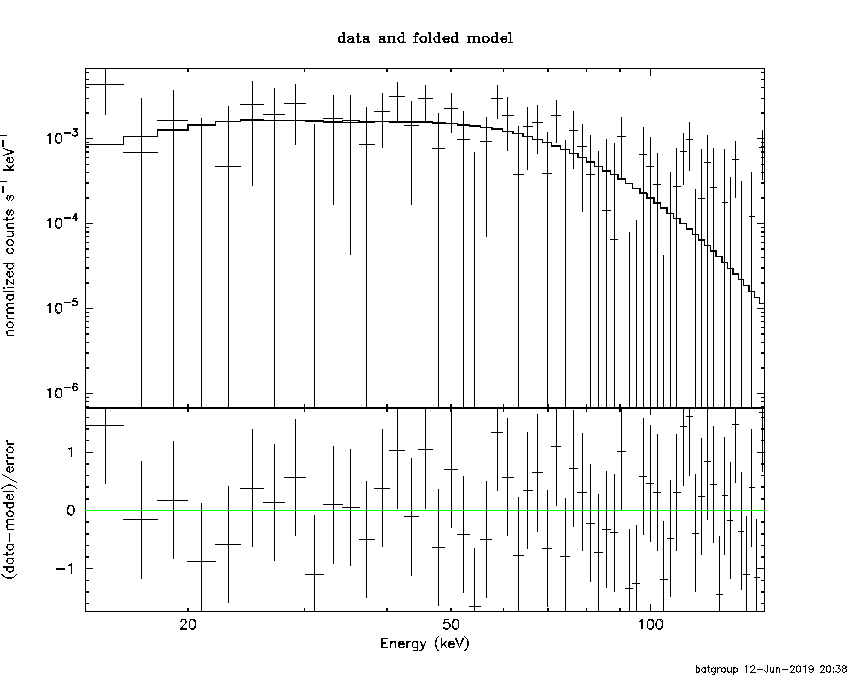```Time interval is from    0.544 sec. to    1.544 sec.
Spectral model in the cutoff power-law:
------------------------------------------------------------
Parameters  : value       lower 90%   higher 90%
Photon index: -1.68460     ( -6.26197   2.78063 )
Epeak [keV] : 68.8866      ( -17.0885 212.75 )
Norm@50keV  : 0.148913    ( -0.155101   77.7859 )
------------------------------------------------------------
#Fit statistic : Chi-Squared =          41.02 using 59 PHA bins.
# Reduced chi-squared =         0.7325 for     56 degrees of freedom
# Null hypothesis probability =   9.333429e-01

Photon flux (15-150 keV)    in 1 sec: 0.741146 ( -0.255471  0.265924 ) ph/cm2/s
Energy fluence (15-150 keV)             : 6.21529e-08 ( -2.17215e-08  2.37186e-08 ) ergs/cm2

```
##### Band function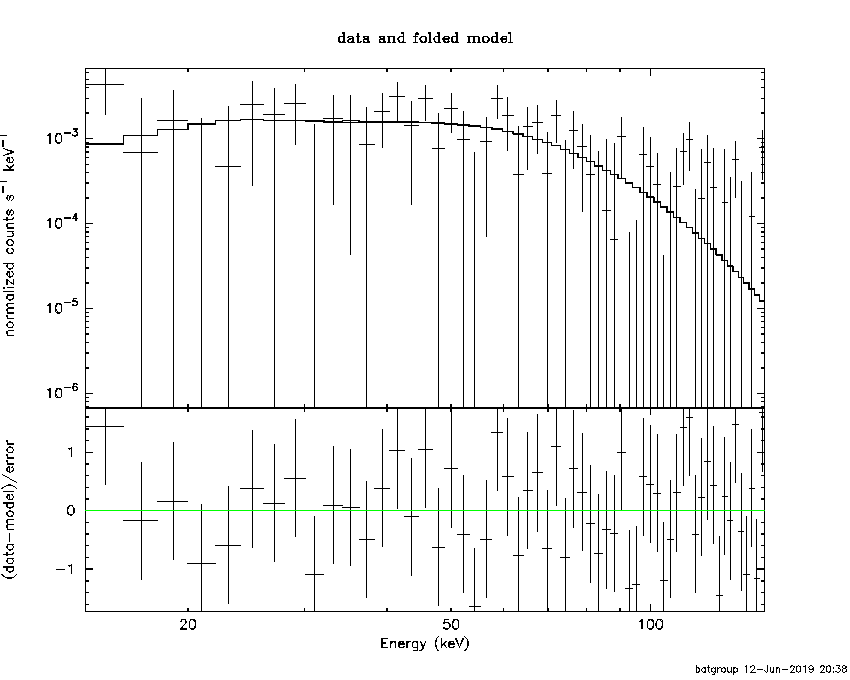```Time interval is from    0.544 sec. to    1.544 sec.

Spectral model in the Band function:
------------------------------------------------------------
Parameters  : value       lower 90%   higher 90%
alpha       : 1.61868   ( -1.5884   3.02739 )
beta        : -10.00000    ( 10   2.97891e-06 )
Epeak [keV] : 69.2203      ( -16.1124 209.282 )
Norm@50keV   : 0.139810    ( -0.0385533   2.14126 )
------------------------------------------------------------
#Fit statistic : Chi-Squared =          41.02 using 59 PHA bins.
# Reduced chi-squared =         0.7459 for     55 degrees of freedom
# Null hypothesis probability =   9.193596e-01

Photon flux (15-150 keV)    in 1 sec:  (    ) ph/cm2/s
Energy fluence (15-150 keV)             : 0 ( 0  0 ) ergs/cm2

```
##### Single BB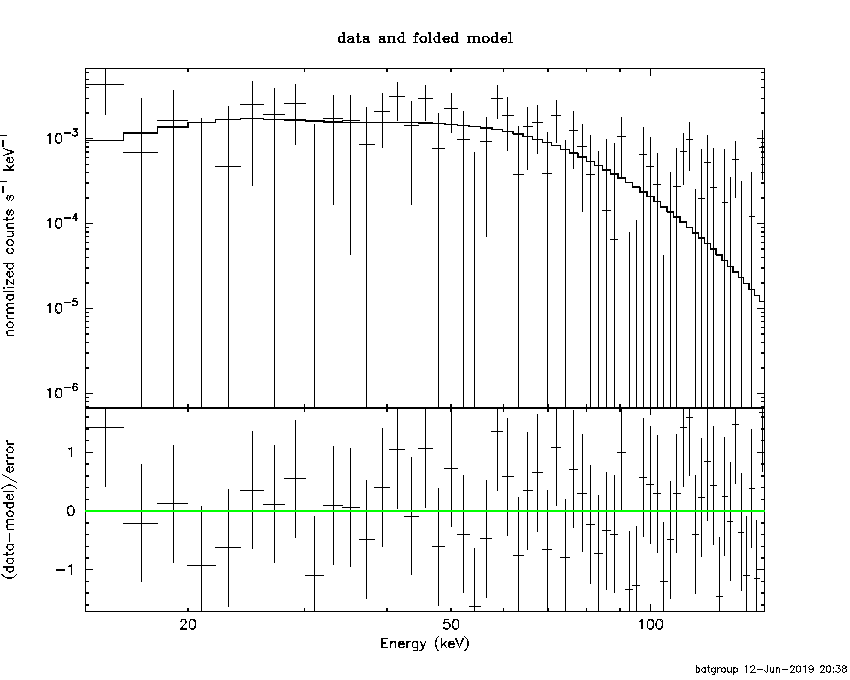```
Spectral model blackbody:
------------------------------------------------------------
Parameters  : value    Lower 90%  Upper 90%
kT [keV]    : 17.9087  (-4.22583  6.42614)
R^2/D10^2   : 5.97986E-02  (-0.0394196   0.0917129)
(R is the radius in km and D10 is the distance to the source in units of 10 kpc)
------------------------------------------------------------
#Fit statistic : Chi-Squared =          41.04 using 59 PHA bins.
# Reduced chi-squared =         0.7201 for     57 degrees of freedom
# Null hypothesis probability =   9.449945e-01

Energy  Fluence     90% Error
[keV]   [erg/cm2]   [erg/cm2]
15- 25  3.846e-09   2.447e-09
25- 50  1.772e-08   9.704e-09
50-150  4.120e-08   2.651e-08
15-150  6.276e-08   3.914e-08
```
##### Thermal bremsstrahlung (OTTB)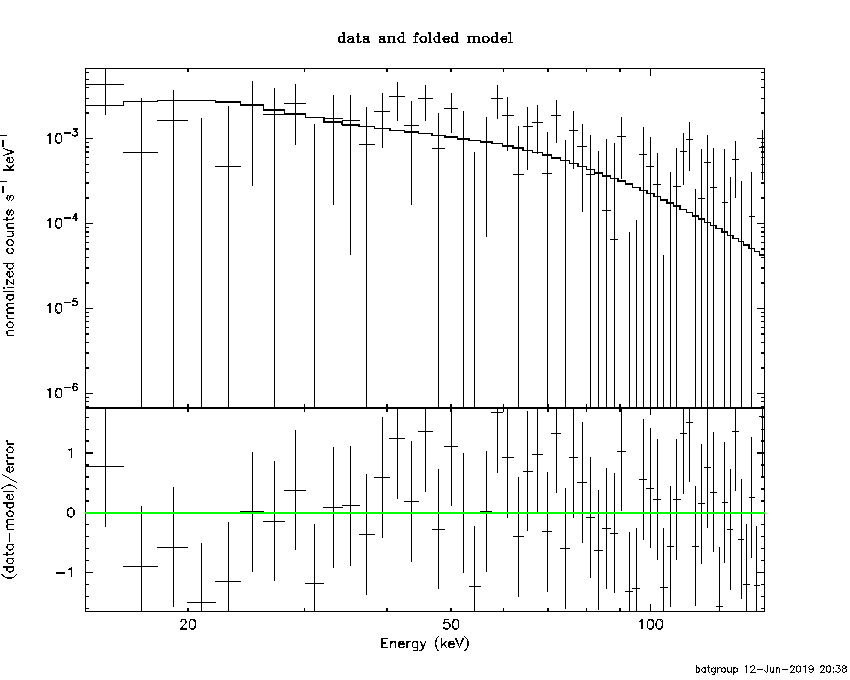```

Spectral model: thermal bremsstrahlung
------------------------------------------------------------
Parameters  : value         Lower 90%    Upper 90%
kT [keV]    : 199.355       (-99.4569      -199.361)
Norm        : 3.49089       (-1.02279     1.02615)
------------------------------------------------------------
#Fit statistic : Chi-Squared =          45.16 using 59 PHA bins.
# Reduced chi-squared =         0.7923 for     57 degrees of freedom
# Null hypothesis probability =   8.714206e-01

Energy  Fluence     90% Error
[keV]   [erg/cm2]   [erg/cm2]
15- 25  8.693e-09   5.365e-09
25- 50  1.672e-08   1.033e-08
50-150  3.667e-08   2.227e-08
15-150  6.209e-08   3.712e-08
```
##### Double BB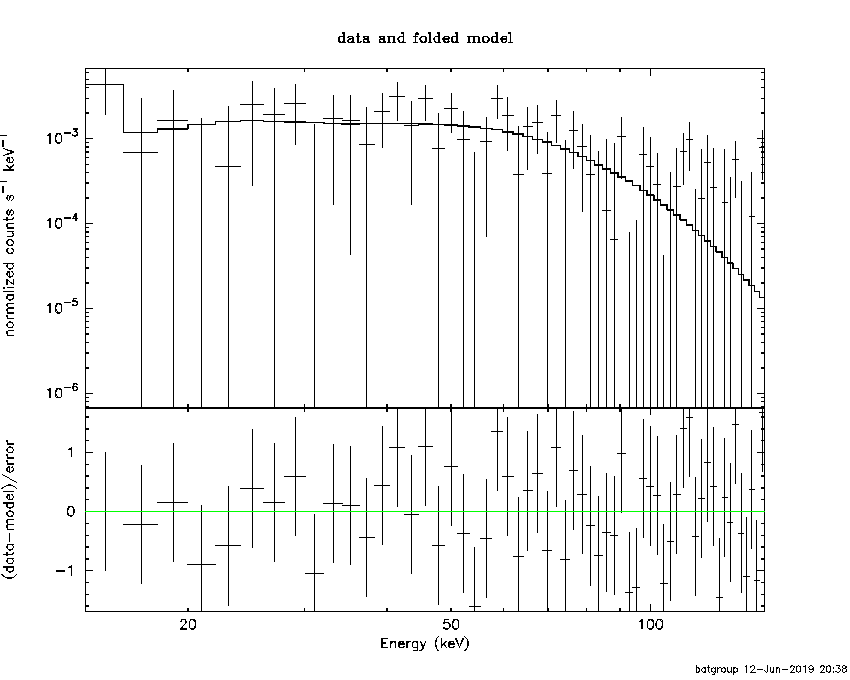```#Fit statistic : Chi-Squared =          39.02 using 59 PHA bins.
# Reduced chi-squared =         0.7165 for     55 degrees of freedom
# Reduced chi-squared =         0.7130 for     55 degrees of freedom
# Reduced chi-squared =         0.7112 for     55 degrees of freedom
# Reduced chi-squared =         0.7103 for     55 degrees of freedom
# Reduced chi-squared =         0.7098 for     55 degrees of freedom
# Reduced chi-squared =         0.7095 for     55 degrees of freedom
# Null hypothesis probability =   9.442178e-01
# Null hypothesis probability =   9.467151e-01
# Null hypothesis probability =   9.480339e-01
# Null hypothesis probability =   9.486753e-01
# Null hypothesis probability =   9.490041e-01
# Null hypothesis probability =   9.491713e-01

Energy  Fluence     90% Error
[keV]   [erg/cm2]   [erg/cm2]
15- 25  4.887e-09   2.447e-09
25- 50  1.679e-08   1.012e-08
50-150  4.210e-08   2.681e-08
15-150  6.378e-08   3.417e-08
```
```Plot creation:
Wed Jun 12 20:38:56 EDT 2019
```